# Hypergeometrical Universe

## Hypergeometrical Gravitational Lensing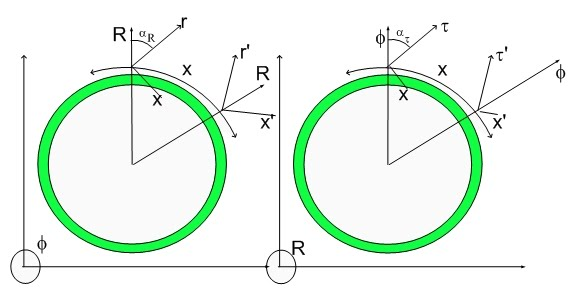Hypergeometrical Gravitational Lensing

There is a famous discovery in Science where the Gravitational Lensing played a strong role…J

Of course, I am referring to the Prediction of Gravitational Lensing by Einstein's General Relativity.

To obtain the predictions of the Hypergeometrical Model for the gravitational refraction of an electromagnetic wave, one has to remember that a Force is represented as a Stress in this model. Acceleration is modeled as a local deformation of the Fabric of Space. This is shown in the equation below: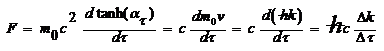Where dτ is equal to cdt, that is, it is a dimensionalized time. The momentum of an electromagnetic wave was represented by hk and its mass by this equation: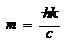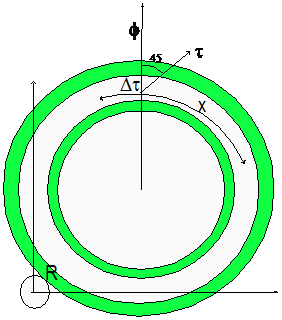Light always travels at 45 degrees with respect to the Fabric of Space as shown above. This means that Gravitation only affects the direction of propagation on the Fabric of Space. That cross-section is shown below:Where the standard phase matching condition (although applied to a single point R=R0 in this case) is shown below: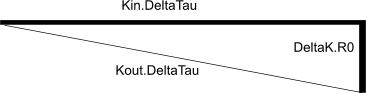Delta Tau is the de Broglie step in the Hypergeometrical Expansion of the Universe. The angle is given by: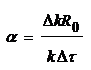The Force can be written in terms of Gravitational fields as: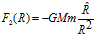Notice that the interaction between an electromagnetic wave doesn't depends upon velocity since the wave cannot be accelerated or decelerates as a particle could.

The equation of motion for an electromagnetic wave is given by:At the position of scattering R=Ro yielding: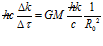Resulting in the diffraction angle: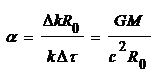Which is the observed Gravitational Lensing. By the way, this result depends upon the de Broglie step DeltaTau.  This means that scattering at R=R0 happens on a single step by that amount.

I didn't see Einstein's derivation but I wonder how could he come up with my de Broglie Universe expansion step...:)  Of course, the observed diffraction is the integral of all diffractive events throughout the Gravitational Lens.

Q.E.D.

Cheers,

MP

Currently unrated

or